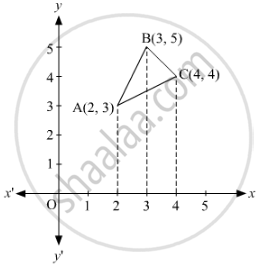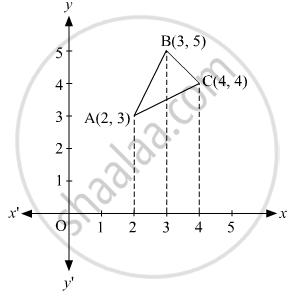# Using Integration, Find the Area of the Triangle Whose Vertices Are (2, 3), (3, 5) and (4, 4). - Mathematics

Sum

Using integration, find the area of the triangle whose vertices are (2, 3), (3, 5) and (4, 4).

#### Solution 1

The Vertices of ΔABC are A (2, 3), B (3, 5), and C (4, 4)Equation of line segment AB is

("y" - 5) = (5-3)/(3-2) ("x" -3)

="y" -5 = 2 ("x" -3)

="y" = 2"x" -1

Equation of line segment BC is

("y" - 5) = (5-3)/(3-4) ("x" -3)

="y" -5 = -1 ("x" -3)

="y" = -"x" + 8

Equation of line segment AC is

("y" - 4) = (4-3)/(4-2) ("x" -4)

="y" -4 = (1)/(2)  ("x" -4)

= "y" = ("x")/(2) + 2

∴ Area of ΔABC = int_2^3 [(2"x" -1) - ("x"/2+2)] d"x" + int_3^4 [(-"x" + 8) - ("x"/2 + 2)] . d"x"

= int_2^3 ((3"x")/2 -3) . d"x" + int_3^4 ((-3"x")/2 + 6) . d"x"

= [(3"x"^2)/(4) - 3"x"]_2^3 + [ (-3"x"^2)/4 + 6"x"]_3^4

= (27/4 - 9) - (3 -6) + (-12 + 24) - (-27/4 + 18)

= (3)/(2) "sq. units".

#### Solution 2

The Vertices of ΔABC are A (2, 3), B (3, 5), and C (4, 4)Equation of line segment AB is

("y" - 5) = (5-3)/(3-2) ("x" -3)

="y" -5 = 2 ("x" -3)

="y" = 2"x" -1

Equation of line segment BC is

("y" - 5) = (5-3)/(3-4) ("x" -3)

="y" -5 = -1 ("x" -3)

="y" = -"x" + 8

Equation of line segment AC is

("y" - 4) = (4-3)/(4-2) ("x" -4)

="y" -4 = (1)/(2)  ("x" -4)

= "y" = ("x")/(2) + 2

Area of ΔABC = int_2^3 [(2"x" -1) - ("x"/2+2)] d"x" + int_3^4 [(-"x" + 8) - ("x"/2 + 2)] . d"x"

= int_2^3 ((3"x")/2 -3) . d"x" + int_3^4 ((-3"x")/2 + 6) . d"x"

= [(3"x"^2)/(4) - 3"x"]_2^3 + [ (-3"x"^2)/4 + 6"x"]_3^4

= (27/4 - 9) - (3 -6) + (-12 + 24) - (-27/4 + 18)

= (3)/(2) "sq. units".

Concept: Area of a Triangle
Is there an error in this question or solution?